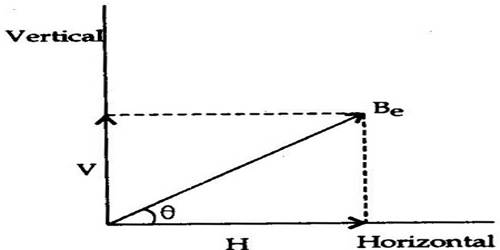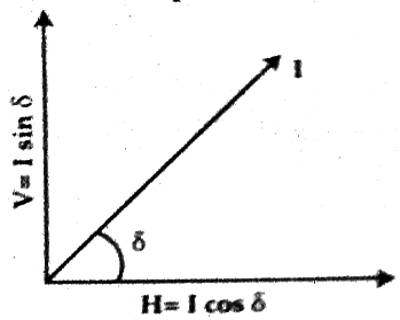# Horizontal Intensity of Earth’s Magnetic Field: Elements of Geomagnetism

Horizontal Intensity of Earth’s magnetic field: Elements of Geomagnetism

Horizontal and vertical components of the earth’s magnetic intensity: The force acting on a unit pole strength of north pole at any place of the earth due to geomagnetism is called the geomagnetic field intensity or total intensity.

A magnet suspended at a place freely through the center of gravity indicates the direction of the magnetic field intensity at that place. Suppose geomagnetic field intensity at a place is I. This intensity I can be resolved into two components — one is the horizontal component H and the other one is the vertical component V (Figure).This horizontal component is called the horizontal intensity H of the geomagnetic field intensity and the vertical component is called the vertical intensity V of the geomagnetic field intensity. Their magnitudes are different at different places of the earth.

Definition: The horizontal component of the geomagnetic held intensity at a place is called the horizontal field intensity of that place and the vertical component is called the vertical field intensity of that place.

According to description,

H = I cos δ … … … (1)

and V = I sin δ … …. … (2)

Here, δ = angle of dip.

By squaring and adding equations (1) and (2) we get,

I2 cos2 δ + I2 sin2 δ = H2 + V2

or, I2 (cos2 δ + sin2 δ) = H2 + V2

or, I2 = H2 + V2

So, I = √( H2 + V2)

Again, dividing equation (2) by equation (1) we get,

I sin δ / I cos δ = V/H

or, tan δ = V/H … …. …. (3)

So, tan-1 (V/H) …. ….. …. (4)

From equation (3) we get, V = H tan δ

or, H/V = 1/tan δ = cot δ

H = V cot δ

[N. B. If instead of I, the geomagnetic field intensity, magnetic field B is used, then in the above equations, I will be changed by B. Then the horizontal component and the vertical component of the magnetic field intensity will be called respectively the horizontal and the vertical components of the magnetic field. In that case, instead of unit Am-1, the unit will be Tesla or Weber/m2.]

Example: Suppose the geomagnetic field intensity of Rajshahi is H = 29 Am-1. By this statement, we mean that at Raishashi (i) the magnitude of the horizontal component of the geomagnetic field intensity, H = 29 Am-1. (ii) The north pole of the unit pole strength will experience a force of 29N along the horizontal of the geomagnetic field. (iii) If the angle of dip of Rajshahi is δ, the vertical intensity of the geomagnetic field, V = 29 tan δ and total intensity I = 29 sec δ.

In the magnetic poles of the earth, there is no horizontal intensity of the geomagnetic field. Its value at the equator is maximum between 30 Am-1 to 32 Am-1. If the angle of dip of Dhaka is 310, then the ratio of the vertical and horizontal Components of the geomagnetic field at Dhaka is equal to tan 31°.